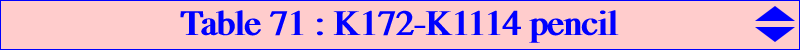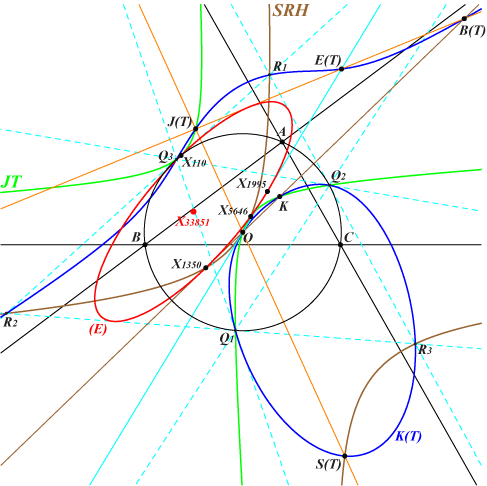K172 and K1114 generate a pencil of cubics of the form K(T) = a^2b^2c^2 K1114 + T 16 ∆^2 K172, where T is a real number or infinity and ∆ is the area of ABC. Obviouly, K(0) = K1114 and K(∞) = K172. Each cubic K(T) passes through : • X(3) with tangent the Euler line unless T = 1/2 for which K(T) is the nodal cubic K1118. Let E(T) be the third point of K(T) on the Euler line hence the tangential of X(3) if T ≠ 1/2. For any T, the polar conic of X(3) is a rectangular hyperbola passing through X3, X2574, X2575, with tangent the Euler line unless T = 1/2 (for which it splits into two perpendicular lines secant at X3). When T = -1/4, it splits into the Euler line and the line at infinity since K(-1/4) = K1117 is a central cubic. • X(6). Let B(T) be the third point of K(T) on the Brocard axis. • the vertices Q1, Q2, Q3 of the Thomson triangle. These points lie on the circumcircle (O), the Thomson cubic K002, the Thomson-Jerabek hyperbola JT which meets K(T) at a sixth point J(T). • the vertices R1, R2, R3 of the tangential triangle of the Thomson triangle. These points lie on SRH (Stammler reflected hyperbola, see preamble of X(33573) in ETC) which meets K(T) at a sixth point S(T). Recall that these two latter triangles are perspective at X(5646), the Lemoine point of the Thomson triangle. Remark : E(T), B(T), J(T) and J(T), S(T), X(3) are triads of collinear points on K(T). When T varies, the line L(T) passing through E(T), B(T), J(T) envelopes an ellipse (E) inscribed in the tangential triangle of the Thomson triangle, passing through X(1995) with tangent the Euler line, X(1350) with tangent the Brocard axis, X(110) with tangent passing through X(23), X(5646). (E) is bitangent at X(110) and X(5646) to JT. The center of (E) is X(33851) on the lines {3, 2854}, {6, 15035}, {20, 14982}, {22, 110}, {67, 10519}, {69, 32233}, {74, 2930}, {113, 29181}, {141, 17702}, {159, 11820}, {182, 12893}, {265, 6698}, {376, 5648}, {511, 1511}, etc. *** Other points on K(T) : • K(T) meets the line at infinity at three points which also lie on some pK(X6, P(T) on the line X2-X51) and on some pK(Ω(T) on the line X4-X39, X4). • K(T) meets (O) again at three other points T1, T2, T3 which also lie on some nK0(X6, R(T) on the line X2-X6) and on some nK0(Ω'(T) on the line X3-X6, X6). The Kiepert parabola is inscribed in this triangle T1T2T3 for any T. Moreover, these points T1, T2, T3 are the antipodes on (O) of the points (apart A, B, C) where the Euler cubic pK(X6, E'(T)) meets (O), see Table 27. The pivot E'(T) is the inverse of E(T) in the circle with center X(23) orthogonal to (O). This is the circle with diameter X(5004)X(5005). • K(T) passes through the vertices S1, S2, S3 of the tangential triangle of the triangle T1T2T3. These points S1, S2, S3 lie on the Stammler hyperbola SH for any T. *** By Poncelet's porism, since Q1, Q2, Q3, T1, T2, T3 lie on (O), the points R1, R2, R3, S1, S2, S3 must lie on a same conic RS which also contains X(2930). The center of RS lies on a rectangular hyperbola homothetic to the Jerabek hyperbola and passing through X3 (T = -1/4), X159 (T = ∞), X3098 (T = 0). RS is a circle if and only if T = 1/2, with center X(33532). This is the circumcircle of the tangential triangle of the Thomson triangle. On the other hand, the triangles Q1Q2Q3 (Thomson) and T1T2T3 must also circumscribe a same conic QT which is also tangent to the trilinear polar of X(524) and whose center is a point on the Euler line of ABC. Its abscissa in (X3,X2) is given by f(T) = (4T + 1) / (4T + 4). Obviously, with T = – 1/4 hence f(T) = 0, K(T) is the central cubic K1117 and the conic has center X(3). With T = – 1, QT is the parabola with focus X(110), directrix the perpendicular at G to the Euler line. The vertices of T1T2T3 are X(110) and the circular points at infinity. *** The following table shows a selection of the most remarkable cubics K(T) with associated points. Those is red were added to ETC on 2019-08-10, those in light blue on 2019-08-21.T / (note) K(T) P(T) Ω(T) R(T) Ω'(T) E(T) B(T) J(T) S(T) ∞ / (0) K172 X(2979) X(3199) X(2) X(32) X(25) X(6) X(154) X(64) 0 K1114 X(2) X(4) X(6) X(6) X(2) X(3098) X(2) X(3) –1 / (1) K1116 X(511) X(232) X(230) X(50) X(23) X(511) X(110) X(10620) –1/4 / (2) K1117 X(5640) X(33843) X(5304) X(33871) X(3) X(1350) X(6) X(1350) 1/2 / (3) K1118 X(7998) X(33842) X(7736) X(33872) X(1995) X(3) X(3) X(11472) / (4) K1119 X(33873) X(33874) X(385) X(33875) X(237) X(5104) X(33876) X(33877) –1/2 K1120 X(3066) X(39) X(7735) X(5063) X(22) X(33878) X(3167) X(12163) 1/8 K1121 X(33879) X(33880) X(14930) X(33881) X(11284) X(31884) X(5646) X(5646) / (5) K1122 X(33852) X(33853) X(33854) X(33882) X(33849) X(33844) X(33883) X(33811) 2 K1123 X(33884) X(33885) X(31489) X(33886) X(14002) X(182) X(7712) X(33887) / (6) K1125 X(34095) X(34096) X(11174) X(34097) X(34098) X(574) X(34099) X(34100)Notes : (0) : K(T) is the only circum-cubic (1) : K(T) is a circular cubic (2) : K(T) is a central cubic (3) : K(T) is a nodal cubic (4) : T = –1 / (4 sin2ω) = – (1 + cot2ω) / 4 (5) : T = – a b c / (8 sa sb sc) (6) : T = 1 / (2 sin2ω) = (1 + cot2ω) / 2First coordinate of mentioned points : P(T) = a^2 b^2 c^2-a^2 (a^2 b^2-b^4+a^2 c^2-b^2 c^2-c^4) T Ω(T) = 2 a^2 b^2 c^2 (a^2+b^2-c^2) (a^2-b^2+c^2)+a^2 (a^2+b^2-c^2) (a^2-b^2+c^2) (a^2 b^2-b^4+a^2 c^2+2 b^2 c^2-c^4) T R(T) = a^2 (a^2+b^2+c^2)-(a-b-c) (a+b-c) (a-b+c) (a+b+c) T Ω'(T) = 3 a^4 b^2 c^2-a^4 (a-b-c) (a+b-c) (a-b+c) (a+b+c) T E(T) = a^2 b^2 c^2+a^2 (a^2+b^2-c^2) (a^2-b^2+c^2) T B(T) = a^2 (a^4+a^2 b^2-2 b^4+a^2 c^2-2 b^2 c^2-2 c^4)+a^2 (a-b-c) (a+b-c) (a-b+c) (a+b+c) T J(T) = a^2 b^2 c^2-a^2 (a^2-b^2-c^2) (b^2+c^2) T-a^2 (3 a^4-2 a^2 b^2-b^4-2 a^2 c^2+2 b^2 c^2-c^4) T^2 S(T) = a^4 b^2 c^2 (a^2-b^2-c^2)-2 a^2 b^2 c^2 (a^2+b^2-c^2) (a^2-b^2+c^2) T-a^2 (a^4-2 a^2 b^2+b^4+2 a^2 c^2+2 b^2 c^2-3 c^4) (a^4+2 a^2 b^2-3 b^4-2 a^2 c^2+2 b^2 c^2+c^4) T^2*** Additional points and loci : • The third point J'(T) of K(T) on the line X(6)-J(T) lies on a hyperbola HR passing through R1, R2, R3 and X(i) for these i : 3, 6, 25, 7669, 8667, 15621, 15624, 33580, 33582, 33861, 33900. It also passes through the infinite points of the circum-hyperbola with perspector X(512) passing through X(2) and X(6). It is the isogonal transform of the line through X(3), X(524) with respect to the tangential triangle R1R2R3 of the Thomson triangle. Its center is : a^4 (a^4 b^4-a^2 b^6-a^4 b^2 c^2-b^6 c^2+a^4 c^4+3 b^4 c^4-a^2 c^6-b^2 c^6) : : , on lines {3, 5969}, {6, 21444}, {32, 1084}, {83, 31639}, {98, 804}, {111, 669}, {160, 5033}, {182, 9145}, {237, 694}, {670, 1078}, {699, 733}, {729, 887}, {888, 9142}, {1634, 5182}, {3124, 11332}, {3228, 5201}, {3398, 15462}, {3511, 5026}, {5038, 30534}, {5104, 32518}, {7793, 25054}, {14910, 16098}, {14931, 32531}, {20998, 23216}. • The tangential B'(T) of B(T) in K(T) lies on a hyperbola HQ passing through Q1, Q2, Q3 and X(i) for these i : 3, 99, 574, 8723, 18773, 18774, 32524, 33705, 33756, 33850, 33929. It also passes through the infinite points of the circum-hyperbola passing through X(98), X(1344), X(1345). It is the isogonal transform of the line through X(2), X(51) with respect to the Thomson triangle Q1Q2Q3. Its center is : a^2 (a^10 b^4-3 a^8 b^6+3 a^6 b^8-a^4 b^10-2 a^10 b^2 c^2+3 a^8 b^4 c^2+a^6 b^6 c^2-5 a^4 b^8 c^2+3 a^2 b^10 c^2+a^10 c^4+3 a^8 b^2 c^4+16 a^6 b^4 c^4-18 a^4 b^6 c^4+6 a^2 b^8 c^4-2 b^10 c^4-3 a^8 c^6+a^6 b^2 c^6-18 a^4 b^4 c^6+6 a^2 b^6 c^6+2 b^8 c^6+3 a^6 c^8-5 a^4 b^2 c^8+6 a^2 b^4 c^8+2 b^6 c^8-a^4 c^10+3 a^2 b^2 c^10-2 b^4 c^10) : : , on lines {237, 33813}, {5027, 9155}. • Remarks : – X(3), J'(T), B'(T) are collinear on K(T). – The third point E'(T) of K(T) on the line X(6)-E(T) also lies on the line S(T)-B'(T). When T varies, the locus of E'(T) is the nodal cubic K1124. – The third point S'(T) of K(T) on the line X(6)-S(T) is the tangential of E(T). When T varies, the locus of S'(T) is a rather complicated quintic passing through X(3) which is a point of inflexion, X(6) which is triple, X(1033), X(1344), X(1345), X(3098), the vertices of the Thomson triangle (which are double), the vertices of the tangential triangle of the Thomson triangle. See table below (and notes above) where most of these points are represented. Those is green were added to ETC on 2019-08-17.T / (note) K(T) J'(T) on HR B'(T) on HQ E'(T) on K1124 S'(T) on quintic ∞ / (0) K172 X(8667) X(33705) X(8667) X(3098) 0 K1114 X(25) X(3) X(154) X(1033) –1 / (1) K1116 X(33900) X(33972) X(33973) ? –1/4 / (2) K1117 X(33861) X(33850) X(1350) X(3) 1/2 / (3) K1118 X(3) X(33929) X(33928) ? / (4) K1119 X(33983) X(99) X(33984) ? –1/2 K1120 X(33974) X(33975) X(33976) ? 1/8 K1121 X(33977) X(33978) X(33979) ? / (5) K1122 X(33985) X(33986) ? ? 2 K1123 X(33980) X(33981) X(33982) ? / (6) K1125 X(6) X(574) ? ?